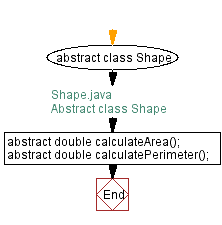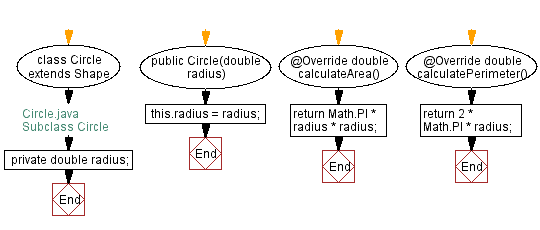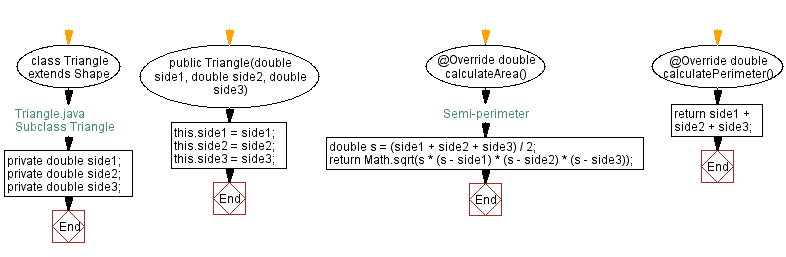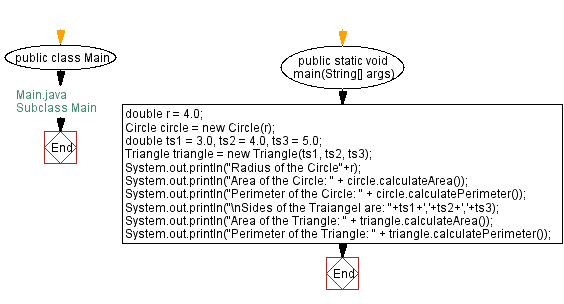﻿ Java - Abstract Shape Class with Circle and Triangle Subclasses

# Java Abstract Classes - Abstract Shape Class with Circle and Triangle Subclasses

## Java Abstract Class: Exercise-2 with Solution

Write a Java program to create an abstract class Shape with abstract methods calculateArea() and calculatePerimeter(). Create subclasses Circle and Triangle that extend the Shape class and implement the respective methods to calculate the area and perimeter of each shape.

In the following program Shape is the abstract base class with two abstract methods: calculateArea() and calculatePerimeter(). The Circle and Triangle classes are subclasses of Shape and provide their own implementations for abstract methods.

Sample Solution:

Java Code:

``````// Shape.java
// Abstract class Shape
abstract class Shape {
abstract double calculateArea();
abstract double calculatePerimeter();
}
```
```
``````// Circle.java
// Subclass Circle
class Circle extends Shape {

}

@Override
double calculateArea() {
}

@Override
double calculatePerimeter() {
return 2 * Math.PI * radius;
}
}
``````
``````// Triangle.java
// Subclass Triangle
class Triangle extends Shape {
private double side1;
private double side2;
private double side3;

public Triangle(double side1, double side2, double side3) {
this.side1 = side1;
this.side2 = side2;
this.side3 = side3;
}

@Override
double calculateArea() {
double s = (side1 + side2 + side3) / 2; // Semi-perimeter
return Math.sqrt(s * (s - side1) * (s - side2) * (s - side3));
}

@Override
double calculatePerimeter() {
return side1 + side2 + side3;
}
}
``````
``````// Main.java
// Subclass Main

public class Main {
public static void main(String[] args) {
double r = 4.0;
Circle circle = new Circle(r);
double ts1 = 3.0, ts2 = 4.0, ts3 = 5.0;
Triangle triangle = new Triangle(ts1, ts2, ts3);
System.out.println("Area of the Circle: " + circle.calculateArea());
System.out.println("Perimeter of the Circle: " + circle.calculatePerimeter());
System.out.println("\nSides of the Traiangel are: "+ts1+','+ts2+','+ts3);
System.out.println("Area of the Triangle: " + triangle.calculateArea());
System.out.println("Perimeter of the Triangle: " + triangle.calculatePerimeter());
}
}
``````

Sample Output:

```Radius of the Circle4.0
Area of the Circle: 50.26548245743669
Perimeter of the Circle: 25.132741228718345

Sides of the Traiangel are: 3.0,4.0,5.0
Area of the Triangle: 6.0
Perimeter of the Triangle: 12.0
```

Flowchart:Java Code Editor:

What is the difficulty level of this exercise?

﻿

## Java: Tips of the Day

DropElements

Removes elements in an array until the passed function returns true. Returns the remaining elements in the array.

Loop through the array, using Arrays.copyOfRange() to drop the first element of the array until the returned value from the function is true. Returns the remaining elements.

```public static int[] dropElements(int[] elements, IntPredicate condition) {
while (elements.length > 0 && !condition.test(elements)) {
elements = Arrays.copyOfRange(elements, 1, elements.length);
}
return elements;
}
```

Ref: https://bit.ly/37YWqzD

We are closing our Disqus commenting system for some maintenanace issues. You may write to us at reach[at]yahoo[dot]com or visit us at Facebook